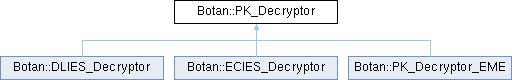Botan 3.2.0 Crypto and TLS for C&
Botan::PK_Decryptor Class Referenceabstract

`#include <pubkey.h>`

Inheritance diagram for Botan::PK_Decryptor:## Public Member Functions

secure_vector< uint8_t > decrypt (const uint8_t in[], size_t length) const

secure_vector< uint8_t > decrypt (std::span< const uint8_t > in) const

secure_vector< uint8_t > decrypt_or_random (const uint8_t in[], size_t length, size_t expected_pt_len, RandomNumberGenerator &rng) const

secure_vector< uint8_t > decrypt_or_random (const uint8_t in[], size_t length, size_t expected_pt_len, RandomNumberGenerator &rng, const uint8_t required_content_bytes[], const uint8_t required_content_offsets[], size_t required_contents) const

PK_Decryptoroperator= (const PK_Decryptor &)=delete

PK_Decryptoroperator= (PK_Decryptor &&) noexcept=default

PK_Decryptor ()=default

PK_Decryptor (const PK_Decryptor &)=delete

PK_Decryptor (PK_Decryptor &&) noexcept=default

virtual size_t plaintext_length (size_t ctext_len) const =0

virtual ~PK_Decryptor ()=default

## Detailed Description

Public Key Decryptor

Definition at line 78 of file pubkey.h.

## ◆ PK_Decryptor() [1/3]

 Botan::PK_Decryptor::PK_Decryptor ( )
default

## ◆ ~PK_Decryptor()

 virtual Botan::PK_Decryptor::~PK_Decryptor ( )
virtualdefault

## ◆ PK_Decryptor() [2/3]

 Botan::PK_Decryptor::PK_Decryptor ( const PK_Decryptor & )
delete

## ◆ PK_Decryptor() [3/3]

 Botan::PK_Decryptor::PK_Decryptor ( PK_Decryptor && )
defaultnoexcept

## ◆ decrypt() [1/2]

 secure_vector< uint8_t > Botan::PK_Decryptor::decrypt ( const uint8_t in[], size_t length ) const

Decrypt a ciphertext, throwing an exception if the input seems to be invalid (eg due to an accidental or malicious error in the ciphertext).

Parameters
 in the ciphertext as a byte array length the length of the above byte array
Returns
decrypted message

Definition at line 21 of file pubkey.cpp.

21 {
23
24 secure_vector<uint8_t> decoded = do_decrypt(valid_mask, in, length);
25
27 throw Decoding_Error("Invalid public key ciphertext, cannot decrypt");
28 }
29
30 return decoded;
31}

Referenced by Botan::KeyPair::encryption_consistency_check().

## ◆ decrypt() [2/2]

 secure_vector< uint8_t > Botan::PK_Decryptor::decrypt ( std::span< const uint8_t > in ) const
inline

Same as above, but taking a vector

Parameters
 in the ciphertext
Returns
decrypted message

Definition at line 96 of file pubkey.h.

96{ return decrypt(in.data(), in.size()); }
secure_vector< uint8_t > decrypt(const uint8_t in[], size_t length) const
Definition pubkey.cpp:21

References decrypt().

Referenced by decrypt().

## ◆ decrypt_or_random() [1/2]

 secure_vector< uint8_t > Botan::PK_Decryptor::decrypt_or_random ( const uint8_t in[], size_t length, size_t expected_pt_len, RandomNumberGenerator & rng ) const

Decrypt a ciphertext. If the ciphertext is invalid (eg due to invalid padding) or is not the expected length, instead returns a random string of the expected length. Use to avoid oracle attacks, especially against PKCS #1 v1.5 decryption.

Definition at line 78 of file pubkey.cpp.

81 {
82 return decrypt_or_random(in, length, expected_pt_len, rng, nullptr, nullptr, 0);
83}
secure_vector< uint8_t > decrypt_or_random(const uint8_t in[], size_t length, size_t expected_pt_len, RandomNumberGenerator &rng) const
Definition pubkey.cpp:78

References decrypt_or_random().

Referenced by Botan::TLS::Client_Key_Exchange::Client_Key_Exchange(), and decrypt_or_random().

## ◆ decrypt_or_random() [2/2]

 secure_vector< uint8_t > Botan::PK_Decryptor::decrypt_or_random ( const uint8_t in[], size_t length, size_t expected_pt_len, RandomNumberGenerator & rng, const uint8_t required_content_bytes[], const uint8_t required_content_offsets[], size_t required_contents ) const

Decrypt a ciphertext. If the ciphertext is invalid (eg due to invalid padding) or is not the expected length, instead returns a random string of the expected length. Use to avoid oracle attacks, especially against PKCS #1 v1.5 decryption.

Additionally checks (also in const time) that: contents[required_content_offsets[i]] == required_content_bytes[i] for 0 <= i < required_contents

Used for example in TLS, which encodes the client version in the content bytes: if there is any timing variation the version check can be used as an oracle to recover the key.

Definition at line 33 of file pubkey.cpp.

39 {
40 const secure_vector<uint8_t> fake_pms = rng.random_vec(expected_pt_len);
41
42 uint8_t decrypt_valid = 0;
43 secure_vector<uint8_t> decoded = do_decrypt(decrypt_valid, in, length);
44
47
48 decoded.resize(expected_pt_len);
49
50 for(size_t i = 0; i != required_contents_length; ++i) {
51 /*
52 These values are chosen by the application and for TLS are constants,
53 so this early failure via assert is fine since we know 0,1 < 48
54
55 If there is a protocol that has content checks on the key where
56 the expected offsets are controllable by the attacker this could
57 still leak.
58
59 Alternately could always reduce the offset modulo the length?
60 */
61
62 const uint8_t exp = required_content_bytes[i];
63 const uint8_t off = required_content_offsets[i];
64
65 BOTAN_ASSERT(off < expected_pt_len, "Offset in range of plaintext");
66
67 auto eq = CT::Mask<uint8_t>::is_equal(decoded[off], exp);
68
70 }
71
74
75 return decoded;
76}
Definition assert.h:50
static Mask< T > is_equal(T x, T y)
Definition ct_utils.h:126
static Mask< T > is_zero(T x)
Definition ct_utils.h:121

## ◆ operator=() [1/2]

 PK_Decryptor & Botan::PK_Decryptor::operator= ( const PK_Decryptor & )
delete

## ◆ operator=() [2/2]

 PK_Decryptor & Botan::PK_Decryptor::operator= ( PK_Decryptor && )
defaultnoexcept

## ◆ plaintext_length()

 virtual size_t Botan::PK_Decryptor::plaintext_length ( size_t ctext_len ) const
pure virtual

Return an upper bound on the plaintext length for a particular ciphertext input length

Implemented in Botan::PK_Decryptor_EME.

The documentation for this class was generated from the following files: